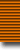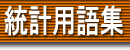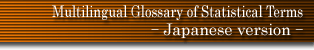### 用語検索

and or
検索単位 単語 フレーズ 完全一致 〜で始まる 〜を含む 〜で終わる 日本語 英語 ドイツ語 フランス語
 日本語 英語 ドイツ語 フランス語
 Bachelier process background information backward equations backward process Bagai's Y1 statistic Bahadur efficiency balanced confounding balanced differences balanced factorial experimental design balanced incomplete block balanced lattice square balanced sample ballot theory Banach's match problem band chart bandit problems bar chart Barnard's Monte Carlo test Bartholomew's problem Bartlett and Diananda test Bartlett relation Bartlett-Lewis model Bartlett's collinearity test Bartlett's decomposition Bartlett's matrix identity Bartlett's test Bartlett's test of second order interaction base base line base period base reversal test base weight basic cell Basu's theorem batch variation Bates-Neyman model battery of tests Baule's equation Bayes' decision rule Bayes' estimation Bayes' factor Bayes' postulate Bayes' risk Bayes' solution Bayes' strategy Bayes' theorem Bayesian confidence intervals Bayesian inference Bayesian intervals Bayesian probability point Beall-Rescia generalisation of Neyman's distribution Bechhofer's indifference zone method behavioural decision function Behrens' method Behrens-Fisher problem Behrens-Fisher test Bellman-Harris process bell-shaped curve Beran's tests Berge's inequality Berksonian line Bernouilli distribution Bernoulli distribution Bernoulli numbers Bernoulli polynomial Bernoulli trials Bernoulli variation Bernoulli walk Bernoulli's theorem Bernstein's inequality Bernstein's theorem Berry's inequality Bessel function distribution Bessel's correction best asymptotically Normal estimator best critical region best estimator best fit best linear unbiased estimator beta binomial distribution beta coefficients beta distribution beta error beta probability plot beta-gamma distribution beta-prime distribution beta-Stacy distribution beta-Whittle distribution better same order bias estimator between-groups variance Bhattacharyya bounds Bhattacharyya's distance bias biased estimator biased sample biased test Bickel-Hodges estimator Bienaymé-Tchebychev inequality bifactor model bilateral exponential bimodal distribution binary experiment binary sequence binary variable Bingham's distribution binomial distribution binomial index of dispersion binomial probability paper binomial variation binomial waiting time distribution bioassay biological assay biostatistics biplot bipolar factor bipolykays Birnbaum-Raymond-Zuckerman inequality Birnbaum's inequality Birnbaum-Saunders distribution Birnbaum-Tingey distribution birth and death process birth process birth rate birth, death and immigration process biserial correlation bispectrum Bissinger distributions bit bivariate beta distribution bivariate binomial distribution bivariate Cauchy distribution bivariate distribution bivariate exponential distribution bivariate F-distribution bivariate gamma distribution bivariate hypergeometric distribution bivariate logarithmic distribution bivariate logarithmic series distribution bivariate multinomial distribution bivariate negative binomial distribution bivariate Normal distribution bivariate Pareto distributiom bivariate Pascal distribution bivariate Poisson distribution bivariate sign test bivariate Student distribution bivariate type II distribution bivariate uniform distribution bivector multinomial distribution Blackwell's theorem Blakeman's criterion block block diagram Blom's method BLUE Blum approximation Blum-Kiefer-Rosenblatt independence test Bock's three component model Bonferroni inequality Boolean factor analysis Boole-Bonferroni-Fréchet inequality Boole's inequality bootstrap methods Borel-Cantelli lemmas Borel-Tanner distribution Borges' approximation Bose distribution Bose-Einstein statistics bounded completeness Bowley index box and whisker plot Box-Cox transformations Box-Jenkins model Box-Müller transformation Box's test Bradford distribution Bradley-Terry model branch and bound methods branching Markov process branching Poisson process branching process branching renewal process Brandt-Snedecor method Bravais correlation coefficient break point breakdown bound Brownian motion process Brown-Mood procedure Brown's method Bruceton method Brunk's test brushing scatterplots Buffon's needle bulk sampling bunch-map analysis Burke's theorem Burkholder approximation Burr's distribution busy period Buys-Ballot table byte